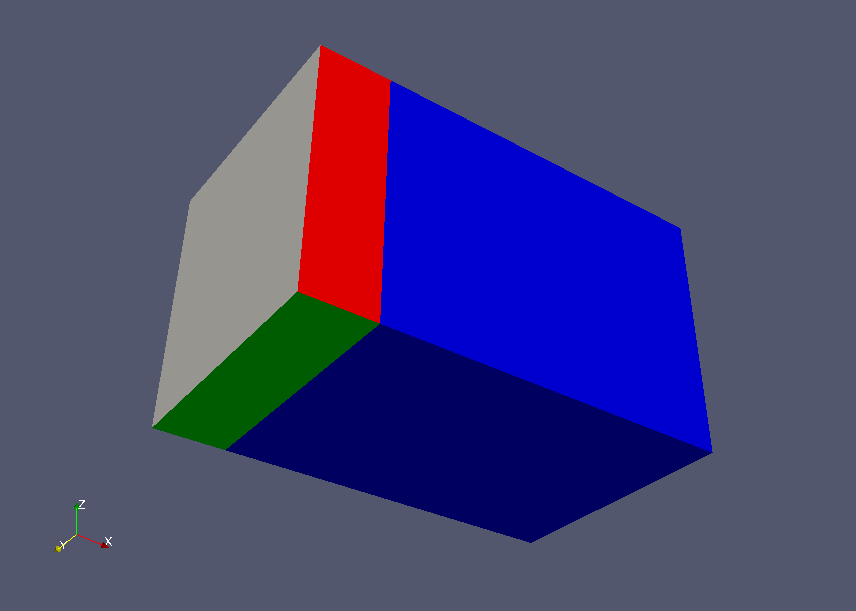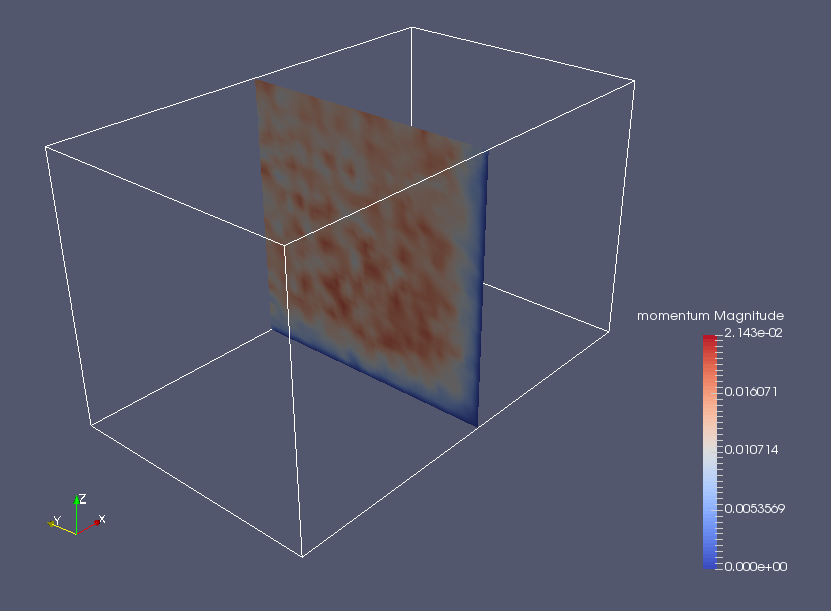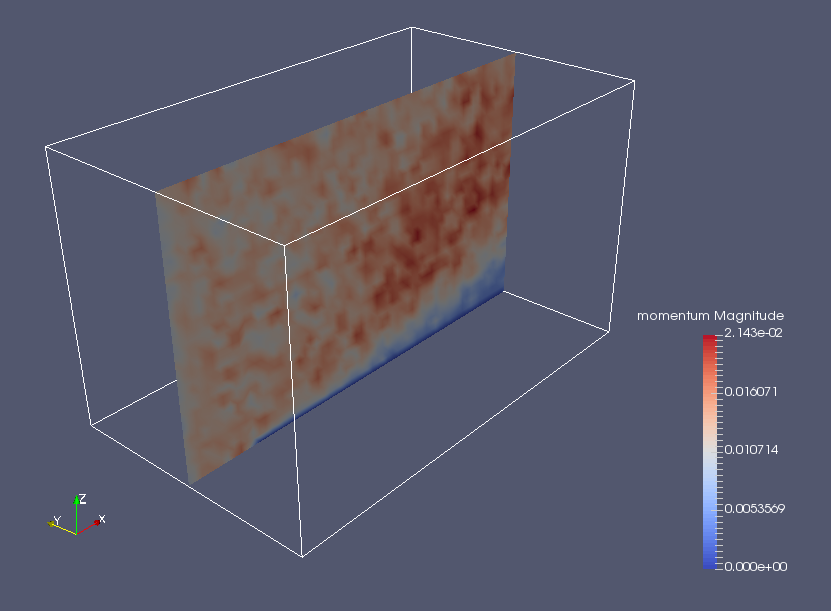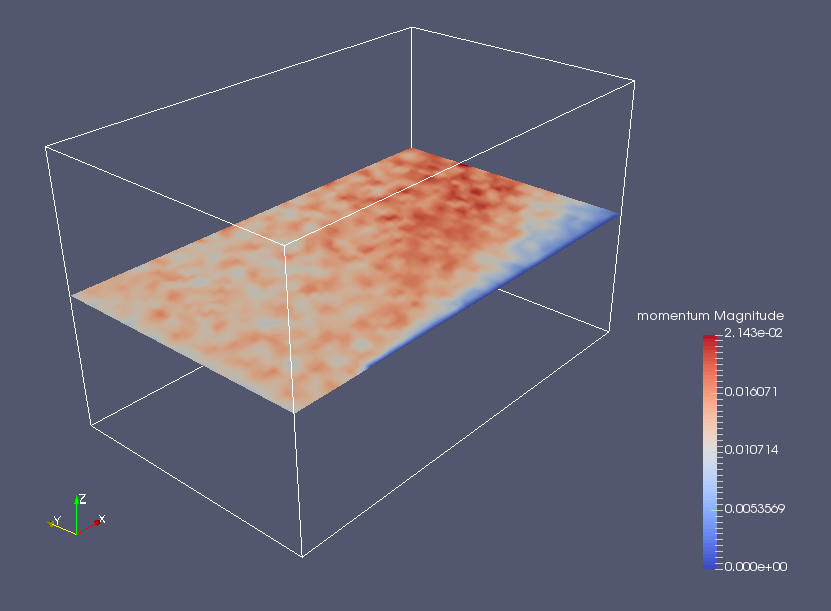﻿ Direct simulation Monte Carlo of supersonic argon gas flow - XSim

# Direct simulation Monte Carlo of supersonic argon gas flow

OpenFOAM 4.x

## Case directory

\$FOAM_TUTORIALS/discreteMethods/dsmcFoam/supersonicCorner

## Summary

We calculate the mixture gas flow of oxygen and nitrogen with the Direct Simulation Monte Carlo (DSMC) method. The Direct Simulation Monte Carlo (DSMC) method was proposed by G. A. Bird in the 1960s to calculate the flow of rarefied gases using the Boltzmann equation.

The properties of the gas are defined in the file "dsmcProperties" in the directory "constant", and the initial state is defined in the file "dsmcInitialiseDict" in the directory "system".

The model is a rectangle with sizes of 30 cm, 18 cm, and 18 cm in the X, Y, and Z axes, respectively, and the minimum faces in the Y and Z coordinates are set to the region "entrance1" (red), "entrance2" (green), and "walls" (blue). The other faces are set as the region "flow".Model geometry

We set a supersonic velocity of (1936, 0, 0) m/s and a temperature of 300 K in the region "flow" as a boundary condition. The region "entrance1" and "entrance2" are symmetric faces, and the region "walls" is a no-slip wall with a temperature of 1000 K.

Each variable in directory 0 is as follows.

• boundaryT - Boundary temperature
• boundaryU - Boundary velocity
• dsmcRhoN - DSMC particles Density
• fD - Force Density (stress vector)
• q - Wall heat flux
• iDof - Density of internal degrees of freedom
• internalE - Internal energy density
• linearKE - Linear kinetic energy density
• momentum - Momentum density
• rhoM - Mass density
• rhoN - Number density
• dsmcSigmaTcRMax - maximum value of "sigmaT × cR" in each cell (see Bird p220)

The calculation result is as follows.Momentum density in the X-axis cross sectionMomentum density in the Y-axis cross sectionMomentum density in the Z-axis cross section

## Commands

cp -r \$FOAM_TUTORIALS/discreteMethods/dsmcFoam/supersonicCorner supersonicCorner
cd supersonicCorner

blockMesh
decomposePar
mpirun -np 4 dsmcInitialise -parallel
mpirun -np 4 dsmcFoam -parallel
reconstructPar -noLagrangian

paraFoam

When combining the divided data in each processors using the command reconstructPar, we added the option "-noLagrangian" to prevent unnecessary Lagrangian data from being combined.

## Calculation time

1 hours 36 minutes 17.2 seconds *4 parallel, Inter(R) Core(TM) i7-2600 CPU @ 3.40GHz 3.40GHz